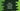# Swift dictionary append method explanation with exampleDictionaries are used to store key-value pairs in Swift. The key is used to access its corresponding value. Using a key, we can edit,delete or update values in a dictionary.

Below is an example of Swift dictionary :

``var number_dict = ["one": 1, "two": 2, "three": 3, "four": 4]``

This dictionary has keys or string type and values of number type.

In this post, I will show you how to append items to a swift dictionary with examples.

## Assign the value to a new key :

This is the easiest way. You can assign a new key to a dictionary with a value :

``dictionary_name[new_key] = value``

If the newkey_ is present in the dictionary, it will update its value with the new value. Else, it will append this key-value pairs to the dictionary. Example :

``````var number_dict = ["one": 1, "two": 2, "three": 3, "four": 4]

number_dict["five"] = 5

print(number_dict)``````

If you run this program, it will print the below output :

``["four": 4, "two": 2, "three": 3, "one": 1, "five": 5]``

### Note :

Note that the datatype of the new key and value should match the datatype of the dictionary keys and values. Else, it will throw an error .

For the below example :

``````var number_dict = ["one": 1, "two": 2, "three": 3, "four": 4]

number_dict = "five"

print(number_dict)``````

It will throw this error :

``error: cannot assign value of type 'String' to subscript of type 'Int'``